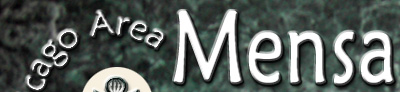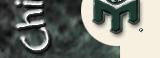# Letter Cubes #1

Rating: easy

### Instructions

Assign the 24 letters A through X to the faces of four cubes so that all of the "words" below can be formed by selecting one face from each cube.

For instance, if the the first word were ABCD, that would tell you that A, B, C, and D were on different cubes. If the second word were ACDH, then you could further deduce that the B and the H were on the same cube.

A D J R

A E J U

B D G U

B I M T

C J M P

D O Q X

E G P U

F I K L

F K O R

F L M V

F L M W

I K N R

N O S X